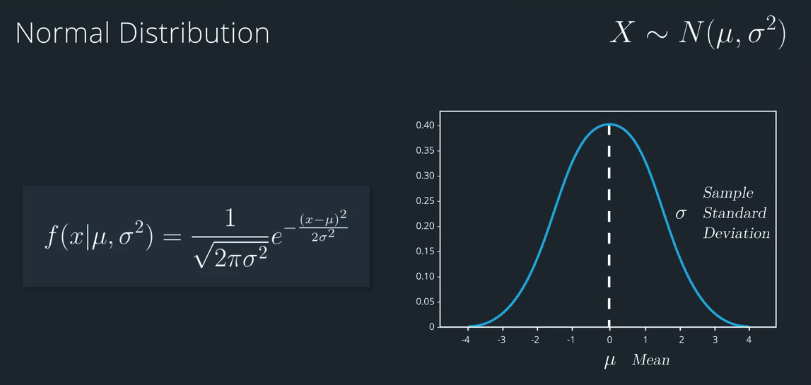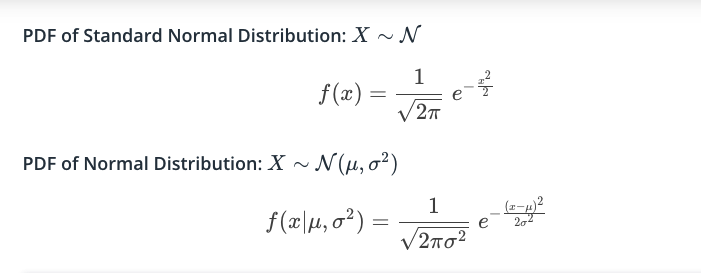# AI For Trading: Parameters of a Distribution (16)

## Normal Distribution### Probability Density Function (Clarification/Correction)

Note that for discrete variables, a probability mass function gives a probability for each value. However, for a probability density function, which describes a continuous variable, the probability is defined as the area under the curve within a range (between two points). For a single point, the area under the curve is actually zero, which is why probabilities for continuous variables are defined within a range instead of a single point.

### Quize:Standard Normal DistributionWhat values of μ and σ make a Normal distribution the same as the Standard Normal distribution?

A : μ=0,σ=0
B: μ=1,σ=1
C: μ=0,σ=1
D: μ=1,σ=e

### Comparing Tests for Normality

There are some visual ways to check if a distribution is normally distributed or not. Recall that normal distributions are symmetric and do not have fat tails (a more formal term for “fat tails” is kurtosis”). Box-whisker plots helps us visually check if a distribution is symmetric or skewed. A histogram lets us check if a distribution is symmetric/skewed, and if it has fat tails. QQ plots help us compare any two distributions, so they can be used to compare distributions other than the normal distribution. If you plot the actual data’s distribution against a theoretical normal distribution, you can decide if the distributions are the same type if the QQ plot produces a fairly straight line.

There are three hypothesis tests that can be used to decide if a data distribution is normal. These are the Shapiro-Wilk test and D’Agostino-Pearson, and the Kolmogorov-Smirnov test. Each of these produce p-value, and if the p-value is small enough, say 0.05 or less, we can say with a 95% confidence that the data is not normally distributed. Shapiro-Wilk tends to perform better in a broader set of cases compared to the D’Agostino-Pearson test. In part, this is because the D’Agostino-Pearson test is used to look for skewness and kurtosis that do not match a normal distribution, so there are some odd non-normal distributions for which it doesn’t detect non-normality, where the Sharpiro-Wilk would give the correct answer.

The Kolmogorov Smirnov test can be used to compare distributions other than the normal distribution, so it’s similar to the QQ plot in its generality. To do a normality test, we would first rescale the data distribution (subtract the mean and divide by its standard deviation), then compare the rescaled data distribution with the standard normal distribution (which has a mean of zero and standard deviation of 1). In general, the Shapiro-WIlk test tends to be a better test than the Kolmogorov Smirnov test, but not in all cases.

So in summary, if you want to be thorough, you can use all three tests (there are even more tests that we haven’t discussed here). If you only want to use one test, use the Shapiro-Wilk test. For a sanity check, visualize your data distribution with a histogram, box-whisker plot, and/or a QQ plot.